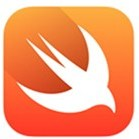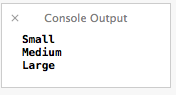### Swift – FunctionsIn the previous post in our series, we looked at control structures in Swift: if statements, switch statements, for loops, and while loops. Today, we are going to look at how to create functions in Swift.

In Objective-C, an instance method would be defined this way:

```- (NSString *)stringByReplacingOccurrencesOfString:(NSString *)target withString:(NSString *)replacement
```

Many newcomers to Objective-C (myself included!) find this syntax to be very confusing. Fortunately, the Swift version of function declaration is much much simpler. Here is a simple example to add two numbers:

```func addTwoNumbers(firstAddend: Int, secondAddend: Int) -> Int {
}
```

You can then call it like this:

```addTwoNumbers(7, 9)
```

The first important thing to note is that the function begins with a func keyword and its implementation body is wrapped in curly braces like C-based methods. Unlike C-based method syntax, the return type isn’t specified before the function name itself. Parameters, while in parentheses like C-based declarations, use a similar syntax to Objective-C with the name:type syntax.

As a side note, just like this Stack Overflow Answer, I’ve always thought that the difference between a function and a method was like this, “A method is on an object. A function is independent of an object. For Java, there are only methods. For C, there are only functions. For C++ it would depend on whether or not you’re in a class”. In Swift, even if a method is in a class (and Swift documentation refers to it as a “method”), it still uses the func keyword. I was thrown off by it a bit and I just wanted to point it out.

You can also accept a dynamic number of parameters in a Swift function. This is often used in string format commands or arguments to be parsed when you start an application. Here, lets just take in a dynamic number of numbers to add together.

```// The declaration
func sumOf(addends: Int...) -> Int {
var sum = 0

}

return sum
}

// All valid ways to call the function
sumOf(1,2,3)
sumOf(7,9)
sumOf(5,4,87,932,144,571)
```

In addition, you can also return multiple values from a function in the form of a Tuple. The declaration is very intuitive – given what we know so far. We just have to change our return type from Int or String into a custom Tuple type, in this case (String, String, String). To access each value, you just use the dot and then the index of the position in the Tuple that you want to access. See below:

```func getSizes() -> (String, String, String) {
return ("Small", "Medium", "Large")
}

var sizes = getSizes()

// Prints Small
println(sizes.0)

// Prints Medium
println(sizes.1)

// Prints Large
println(sizes.2)
```

The console output is then:In Swift, functions are first class citizens and can be passed in as arguments, or returned as values. This can be used like the block syntax in Objective-C, or to work in Swift as a functional programming language paradigm. Here is an example of a function that returns another function, which can then be used later.

```// Here is our function, you see that the return is
// a function that takes two ints and returns another int
func createSummingFunction() -> (Int, Int) -> Int {

// I've declared a function scoped inside the parent function
// Note that it has the same signature that we need to return
}

// Return the function like any other value
return sumIt
}

// Assign the function to a new variable
var mySummer = createSummingFunction()

// Use the variable like it was the original function anyway
// Sets sum = 84
var sum = mySummer(7, 77)
```

Here is an example of a function that takes another function:

```// Created a function that takes an array of integers as well as a function
// and it returns an array of integers.  The function takes in an Int and returns an Int
func doSomethingToNumbers(list: [Int], operation: Int -> Int) -> [Int] {

// Declare our Int array to return
var returnValues = [Int]()

// For every number we passed in
for number in list {
// pass that number to the passed in function ...
var newNumber = operation(number)

// ... and put the new value in the return array
returnValues.append(newNumber)
}

// Return our Int array
return returnValues
}

// We'll use the same array both times
var ourNumberList = [1,2,3]

// Declare a "Plus 1" function that meets the criteria
func plusOne (number: Int) -> Int {
return number + 1
}

// Declare a "Times 2" function that meets the criteria
func timesTwo (number: Int) -> Int {
return number * 2
}

// Added Array now contains [2,3,4]

// Multiplied Array now contains [2,4,6]
var multipliedArray = doSomethingToNumbers(ourNumberList, timesTwo)
```

Lastly, instead of a predefined function, I can also pass in an anonymous function. This allows us to get the same feature set as Objective-C’s block syntax. Assuming we still have our doSomethingToNumbers function and our ourNumberList array in scope, I could do this:

```// Squared Array now contains [1,4,9]
var squaredArray = doSomethingToNumbers(ourNumberList, {(number: Int) -> Int in
return number*number
});
```

To pass in a function as a closure like that, you wrap the entire thing in curly braces, then you define the signature. Instead of using curly braces again to define the body of the function, you use the “in” keyword and define the body of your closure, like I did in the example above.

As you can see, Swift functions are simple and powerful with a syntax that is much easier to understand and read than Objective-C syntax. With very little effort, you should be able to grasp the syntax and start building reusable blocks of code. In the next post in this series, we’ll make a simple “Hello, World” application with Swift.

## One Comment#### Swift – Our First iOS Application  on July 26th, 2014

[…] Last time, we looked at creating functions in Swift. This time, we are going to stop being theoretical and playing in the playground and make an honest to goodness iOS application. […]